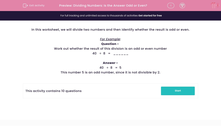# Dividing Numbers: Is the Answer Odd or Even?

In this worksheet, student identify whether the answer to a division problem is an odd or even number.This content is premium and exclusive to EdPlace subscribers.Key stage:  KS 2

Curriculum topic:   Maths and Numerical Reasoning

Curriculum subtopic:   Mixed Problems

Difficulty level:#### Worksheet Overview

In this worksheet, we will divide two numbers and then identify whether the result is odd or even.

For Example:

Question -
Work out whether the result of this division is an odd or even number

40   ÷  8   =   ______

40   ÷  8   =  5

This number 5 is an odd number, since it is not divisible by 2.

### What is EdPlace?

We're your National Curriculum aligned online education content provider helping each child succeed in English, maths and science from year 1 to GCSE. With an EdPlace account you’ll be able to track and measure progress, helping each child achieve their best. We build confidence and attainment by personalising each child’s learning at a level that suits them.

Get started